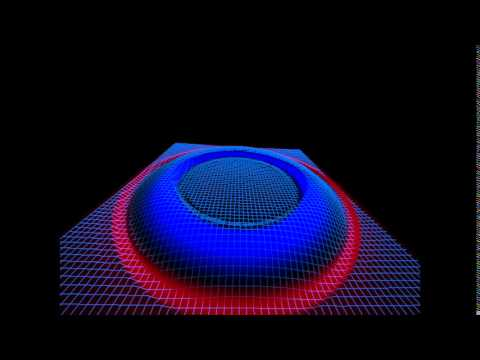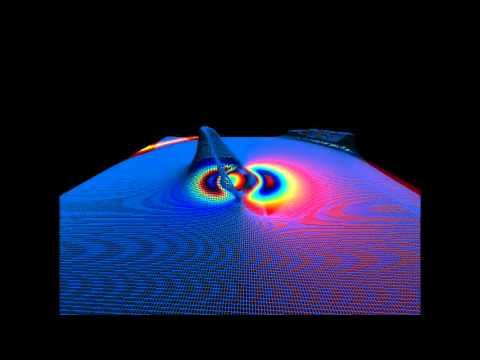Home C++ Lua GitHub Games Math Myself Contact
Hydrodynamics in OpenCL
1D, 2D, 3D CFD via OpenCL - GitHub
• Various flux limiter.
• Mirror, periodic, and free-flow boundary conditions per-side of the grid.
• Self-gravitation via discrete Poisson equation.
• Equations:
• Euler compressible fluid equations. Maxima Worksheet.
• special-relativistic Euler compressible fluid equations.
• Maxwell's equations.
• Bona-Masso hyperbolic conservation law representation of ADM equations.
Explicit Integration Solvers:
• Burgers
• HLL
• HLLC
• Roe

1D, 2D, and 3D CFD - GitHub
• Various flux limiters.
• Euler, Runge-Kutta-2, Runge-Kutta-4, and Iterative Crank-Nicolson-3 explicit integration.
• Mirror, periodic, and free-flow boundary conditions.
• Compressible Euler fluid equation simulation with the following:
Initial Conditions:
• Sod shock tube test initial conditions
• Sedov blast test initial conditions
• simple bell curve perturbation
Solvers:
• Burgers
• Roe

### Bona-Masso ADM Numerical Relativity Results:

Gravitational wave extrinsic curvature from a Schwarzschild equation-of-structure:Slow and stable alcubierre warp bubble collapse:Sources:
Hydrodynamics II: Numerical methods and applications
I do like CFD's Free CFD Codes
"Riemann Solvers and Numerical Methods for Fluid Dynamics," Toro
http://people.nas.nasa.gov/~pulliam/Classes/New_notes/euler_notes.pdfKelvin-Hemholtz instabilityKelvin-Hemholtz instabilityself-gravitating fluid Rayleigh-Taylor instabilityself-gravitating fluid Rayleigh-Taylor instabilityself-gravitating fluid Rayleigh-Taylor instabilityself-gravitating fluidself-gravitating fluidself-gravitating fluidself-gravitating fluid Rayleigh-Taylor instabilityself-gravitating fluid Rayleigh-Taylor instabilityself-gravitating fluid Rayleigh-Taylor instabilityself-gravitating fluidself-gravitating fluid Rayleigh-Taylor instabilitysquare shock wavesquare shock wavesquare shock wavesquare shock wavesquare shock wavesquare shock wave